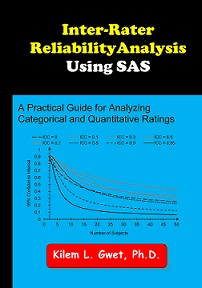﻿ Inter-Rater Reliability

# Inter-Rater Reliability using the SAS System by Kilem Li Gwet, PhD

I wrote this book, primarily to assist researchers and students with the calculation of various inter-rater reliability coefficients using the SAS system. For categorical ratings, you will learn how to use SAS for computing various chance-corrected agreement coe cients (CAC) such as Cohen's kappa, Gwet's $\mbox{AC}_1$/$\mbox{AC}_2$, Krippendorff's alpha and many others. For quantitative ratings, you will learn how SAS can be used to compute different variants of the Intraclass Correlation Coefficient (ICC).

Review the Errata webpage of this book for errors reported by other readers and by myself.

### Inter-Rater Reliability with SAS by Kilem L. Gwet, PhDChance-corrected Agreement Coefficients (CAC)

CAC SAS/IML Librairies

CAC Programs in Chapters 2 & 3

• prg2contingency.sas: Basic SAS program for computing the kappa coefficient based on a
contingency table.
• prg2raw.sas: Basic SAS program for computing the kappa coefficient based on raw scores.
• prg2xtab3cats.sas: SAS program that fixes the diagonal and imbalance problems.
• prg2raw2r.sas: SAS program that xes the diagonal and imbalance problems.
• prg2freq.sas: The FREQ procedure with an unbalanced rating table.
• prg2xtabiml.sas: SAS program that computes alternative agreement coefficients from a contingency table, using SAS/IML functions.
• prg3raw.sas: Using SAS/IML functions in agreecoeff3raw.sas for performing an unweighted analysis of raw ratings.
• prg3dist.sas: Using SAS/IML functions in agreecoeff3dist.sas for analyzing raw data
• prg3wted.sas: Using SAS/IML functions in agreecoeff3raw.sas for computing weighted agreement coefficients based on ordinal weights.
• prg3missfreq.sas: Using the MISSING option in the TABLES Statement of the FREQ procedure for handling missing ratings.
• prg3miss2raters.sas: Calculating several agreement coefficients based on 2 raters with missing ratings - An alternative to the FREQ procedure.
• prg3magree.sas: SAS program that computes Fleiss' generalized kappa and
Gwet's AC1 using the MAGREE.SAS SAS macro.
• prg3raw.sas: Using SAS/IML functions in agreecoeff3raw.sas for performing an unweighted analysis of raw ratings.
• prg3raw10x4.sas: Using SAS/IML functions in agreecoeff3raw.sas for performing an unweighted analysis of Table 3.1 ratings.
• prg5pttest.sas: SAS program for testing the difference between two agreement coefficients for statistical significance
• prg5benchmark.sas: SAS program for computing cumulative membership probabili-
ties to benchmark agreement coefficients.

Intraclass Correlation Coefficients (ICC)

1) ICC SAS/IML Librairies

• icc1factor.sas - Intraclass Correlation Coefficients (ICC) for one-factor models.
• icc2x3.sas - Intraclass Correlation Coefficients (ICC) for two-factor models.

2) ICC Programs in Chapter 4

• prg4shrout.sas: Using the INTRACC.SAS SAS macro and Table 2 of Shrout & Fleiss (1979), for computing intraclass correlation coefficients.
• prg4icc1.sas: Calculating Intraclass Correlation Coefficients (ICC) under the one-factor ANOVA model
• prg4icc2.sas: Calculating Intraclass Correlation Coe cients (ICC) under the
two-way ANOVA model with random effects (Model 2).
• prg4icc3.sas: Calculating Intraclass Correlation Coe cients (ICC) under the
two-way ANOVA model with mixed effects (Model 3).
• prg4finn.sas: SAS program for calculating Finn's agreement coefficient and the associated p-value.